06 Mar 2023

Posted on:

04 Mar 2023

0

# Why the output varies in these function declatarions?

In the downloadable file "More on_function" we have these pieces of code (exit is what we obtain where we execute it):

FIRST CASE

def lengthen_list(n,my_list = [1,2,3]):
my_list.append(n)

return my_list

x = lengthen_list(4)
print(x)
#exit is [1,2,3,4]
x = lengthen_list(4)
print(x)
#exit is [1,2,3,4,4]
x = lengthen_list(4)
print(x)
#exit is [1,2,3,4,4,4]

SECOND CASE

def lengthen_list_2(n,my_list = None):
if my_list == None:
my_list = [1,2,3]
my_list.append(n)
return my_list

y = lengthen_list_2(4)

print (y)
#exit is [1,2,3,4]
y = lengthen_list_2(4)

print (y)

#exit is [1,2,3,4]
y = lengthen_list_2(4)

print (y)

#exit is [1,2,3,4]

Why do we have a difference in exits? It seems that in the second case, my_list gets erased each time we run the code, and this doesn't happen in the first case.

Instructor
Posted on:

06 Mar 2023

0

Hey Ariel,

Thank you for reaching out and thank you for the great question!

This is a Python's peculiarity and is important to keep it in mind when defining functions. It demonstrates the difference in behavior between mutable and immutable objects when applied to function definitions.

A mutable object can change its value while keeping its ID number (you can print out the ID of an object by typing `id(object_name)`). Such are for example lists and dictionaries. Below, you can see an example of a mutable object changing its value and, at the same time, keeping its ID: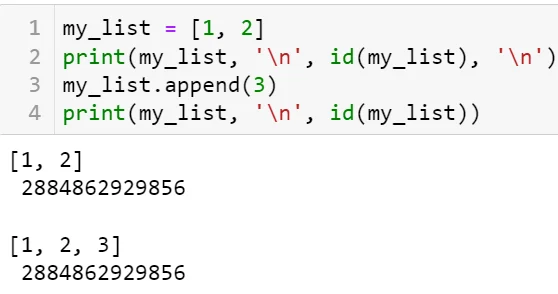An immutable object, on the other hand, can't change its value and keep its ID at the same time. Below is an example: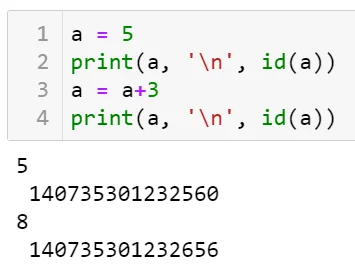In the More_on_functions.py script, the functions `sum_and_mult(a, b)` and `add1(var_3, var_4)` demonstrate how immutable objects behave when serving as arguments of a function. For example, you can see that the variable `var_3 `inside the function and the variable `var_3` outside the function bear different values since they are different objects (have different IDs):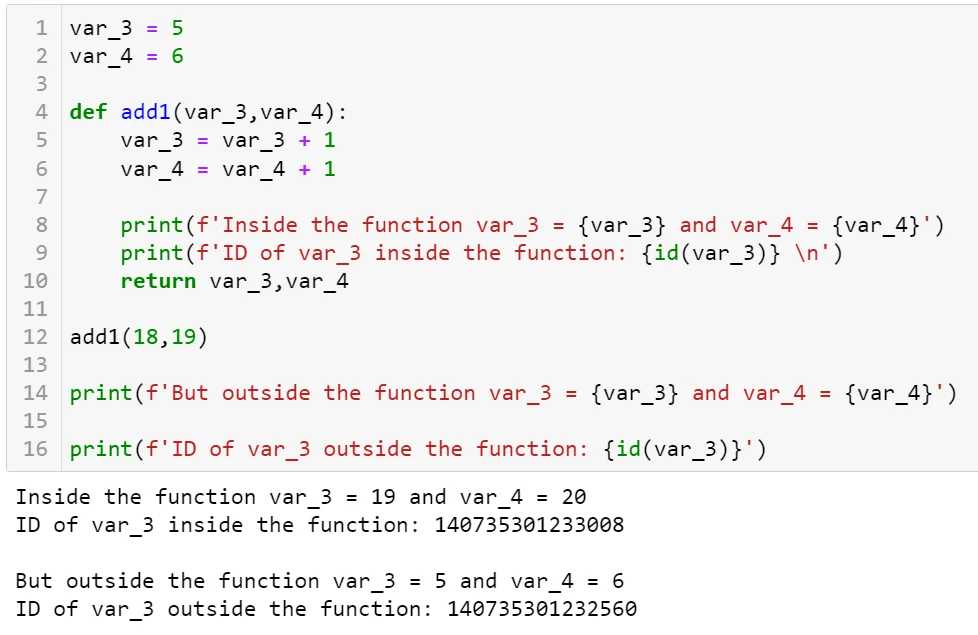In contrast, the functions `lengthen_list(n,my_list = [1,2,3])` and `lengthen_list_2(n,my_list = None)` demonstrate how mutable objects behave when serving as arguments of functions. In this example, we can see that `my_list` and `x` are, in fact, the same object (have the same IDs):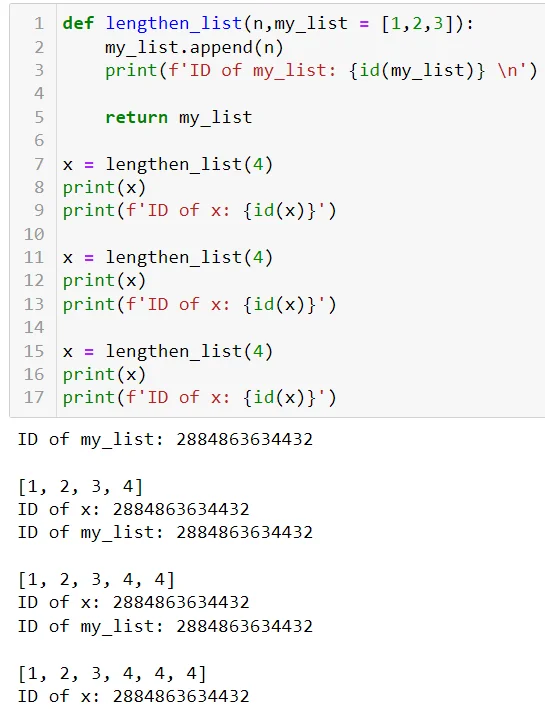If you want to avoid this accumulation and append to a new list each time, then you'd need to define the function as done in `lengthen_list_2(n,my_list = None)`: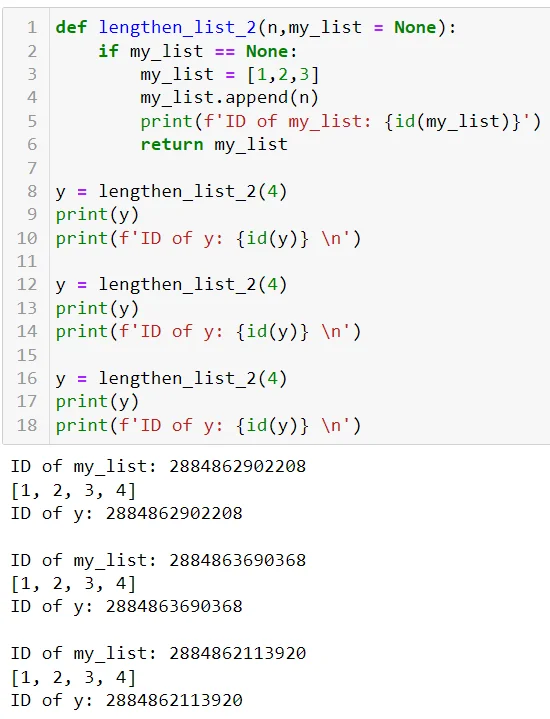Hope this clears up the confusion!

Kind regards,

365 Hristina